Courses

# Competition Level Test: Inverse Trigonometry- 3

## 20 Questions MCQ Test Mathematics For JEE | Competition Level Test: Inverse Trigonometry- 3

Description
This mock test of Competition Level Test: Inverse Trigonometry- 3 for Class 12 helps you for every Class 12 entrance exam. This contains 20 Multiple Choice Questions for Class 12 Competition Level Test: Inverse Trigonometry- 3 (mcq) to study with solutions a complete question bank. The solved questions answers in this Competition Level Test: Inverse Trigonometry- 3 quiz give you a good mix of easy questions and tough questions. Class 12 students definitely take this Competition Level Test: Inverse Trigonometry- 3 exercise for a better result in the exam. You can find other Competition Level Test: Inverse Trigonometry- 3 extra questions, long questions & short questions for Class 12 on EduRev as well by searching above.
QUESTION: 1

### The set of values of ‘a’ for which x2  + ax + sin–1 (x2  – 4x + 5) + cos–1 (x2  – 4x+5 ) = 0 has at least one solution is

Solution: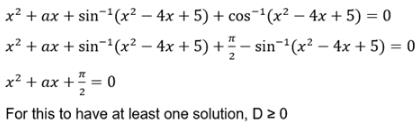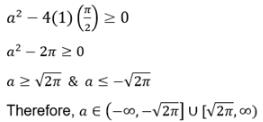QUESTION: 2

### The value of x for which sin [cot–1(1+x)] = cos(tan–1x)

Solution:

sin[cot−1(x+1)] = cos(tan−1x)
⇒ sin[sin^−1(1/√1+(x+1)2)] = cos[ cos−1(1/√1+x2)]
⇒ 1/(√1+(x+1)2) = 1/(√1+x2)
⇒ 1/(x2+2x+2) = 1/(√x2+1)
⇒ x2+2x+2 = x2+1
⇒ 2x + 2 = 1
⇒ 2x = 1 − 2
⇒ 2x = −1
⇒ x = −1/2

QUESTION: 3

### The value of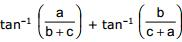, if ∠C = 900 in triangle ABC, is

Solution:

a2 + b2 = c2
tan-1 A + tan-1 B = tan(A + B)/(1 - AB)
=> tan-1 (a/(b+c)) + tan-1 (b/(c+a))
tan-1 [(a/(b+c) + b/(c+a))/(1 - ab/(b+c)(c+a))]
=> tan-1(ac + a2 + b2 + bc)/(bc + ab + c2 + ac - ab)
= tan-1 (ac + bc + a2 + b2)/(ac + bc + c2)
= tan-1 (ac + bc + c2)/(ac + bc + c2)  As we know that {a2 + b2 = c2}
= tan-1(1)
= π/4

QUESTION: 4

The complete solution set of the inequality [cot–1 x]2  – 6[cot–1 x] + 9 ≤0 is (where [ * ] denotes the greatest integer function)

Solution: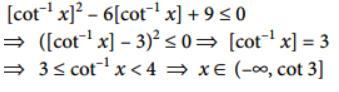QUESTION: 5

If u =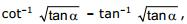then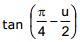is equal to

Solution:
QUESTION: 6

The solution of the equation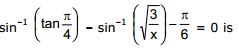Solution:

sin-1(tanπ/4) - sin-1(√3/x) - /6 = 0
sin-1(1) - π/6 = sin-1(√3/x)
π/2 - π/6 = sin-1(√3/x)
2π/6 = sin-1(√3/x)
π/3 = sin-1(√3/x)
⇒. sin(π/3) = (√3/x)
⇒ (√3/2) = (√3/x)
⇒ (x)½ = 2
x = 4

QUESTION: 7

The number of solution(s) of the equation, sin–1x + cos–1 (1 – x) = sin–1 (– x), is/are

Solution:

sin-1 x + cos-1(1-x) = -sin-1 x
cos-1(1-x) = -2sin-1 x
(1-x) = cos(-2sin-1 x) ……………………….(1)
Let 2sin-1 x = t
x= sin t/2
Cos t = 1 - 2sin2 (t/2)
⇒ 1 - 2x^2
From eq(1), we get ⇒ 1- x= 1-2x2
2x2 - x = 0
x(2x - 1) = 0
x = 0,½
sin-1 + cos-1(1-x) = sin-1(-x)
0 + 0 + 0 = 0
π/6 + π/3  ⇒ π/2
sin(-x) = -π/6 not equal to π/2
x = 0, therefore it has 1 solution.

*Multiple options can be correct
QUESTION: 8

If sin-1 x + sin-1 y + sin-1z =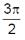, then

Solution:

sin-1 x implies [-π/2, π/2]
= sin-1 x + sin-1 y + sin-1 z = 3π/2
sin-1x = π/2      sin-1y = π/2    sin-1 z = π/2
x = 1       y = 1    z = 1
(A) = 1+1+1 -(9/(1+1+1))   = 3 - 9/3
⇒ 3 - 3 = 0
(B) = 1+1+1-1-1-1 = 0

*Multiple options can be correct
QUESTION: 9

If α satisfies the inequation x2 – x – 2 > 0, then which of the following exists

Solution:

α^2 - α -2 > 0
α^2 - 2α + α -2 > 0
α(α-2) +1(α-2) > 0
(α-2) (α+1) > 0
(-∞, -1) U (2,∞)
Therefore α exist for sec-1α and cosec-1 α

QUESTION: 10

If ƒ(x) = -2x+8, then f-1(1) =

Solution:
*Multiple options can be correct
QUESTION: 11

If the numerical value of tan (cos–1 (4/5) + tan–1 (2/3)) is a/b then

Solution: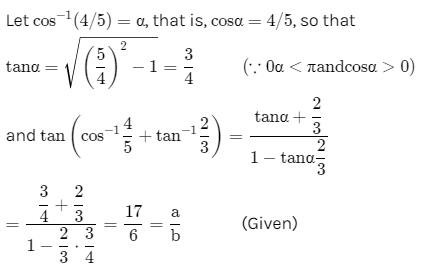Therefore a + b = (17 + 6) = 23
a - b = (17-6) = 11
3b = a + 1
=> 3(6) = 18,
a + 1 => 17 + 1 = 18

*Multiple options can be correct
QUESTION: 12

The value of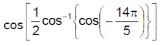is

Solution:

cos(−14π/5)=cos.14π/5=cos.4π/5
Hence, cos.1/2cos−1(cos.4π/5)
=cos.2π/5

*Multiple options can be correct
QUESTION: 13

If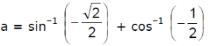and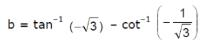,  then

Solution:
*Multiple options can be correct
QUESTION: 14

Let f(x) = sin–1 x + cos–1 x. Then π/2 is equal to

Solution:

We know that for the relation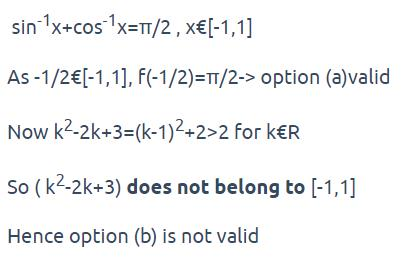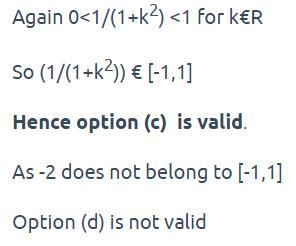*Multiple options can be correct
QUESTION: 15

If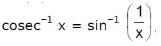, then x may be

Solution:
*Multiple options can be correct
QUESTION: 16

If 0 < x < 1, then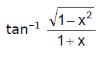is equal to

Solution:
*Multiple options can be correct
QUESTION: 17

α, β and γ are three angles given by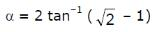,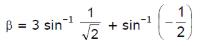and γ = cos-1 1/3. then

Solution:

α = tan-1((2)½ - 1) = 2tan-1 tanπ/8
=2 * π/8
=> π/4 = cos-1(1/(2)½)
β = 3(π/4) - π/6 = 7π/12
Therefore, β > α
⅓ < 1/(2)½
=> cos-1(⅓) > cos-1(/(2)½) = π/4
So, γ > α

*Multiple options can be correct
QUESTION: 18

For the equation 2x = tan (2 tan–1 a) + 2 tan (tan–1 a + tan–1 a3), which of the following is invalid ?

Solution:

2x = tan(2tan-1 a) + 2tan(tan-1 a + tan-1 a3)
= 2 tan(tan-1 a)/[1-{tan(tan-1 a)}2] + 2tan (tan-1 (a+a3)/(1-a*a3))
=2a/(1-a2) + 2(a+a3)/(1-a*a3)
= [2a + 2a3 + 2a + 2a3]/(1-a2)(1+a2)
= 4a/(1-a2)(1+a2)
= 4a(1+a2)/(1-a2)(1+a2)
2x = 4a/(1-a2)
2x(1-a2) = 4a
x = 2a/(1-a2)
=> a2x + 2a - x = 0

QUESTION: 19

cos–1 x = tan–1 x then

Solution:

Let cos−1 x = tan−1 x = y
⇒x=cosy and tany=x
Now,tany=x
siny/cosy = x
⇒(siny)/x=x
⇒siny=x2
siny=cos2y
⇒siny=(1−sin2y)
⇒sin2y+siny−1 = 0
siny = −1±√(1)2−4×1×(−1))/(2 * 1)
​⇒siny=(−1±√5)/2
∵siny∈[−1,1]
∴siny=(√5−1)/2
⇒sin(cos^−1x) = (√5−1)/2

QUESTION: 20

The sum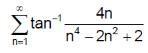is equal to

Solution:

Σ(n = 1) tan-1{4n/(n4 - 2n + 2)}
=  tan-1{4n/(1 + (n2 - 1)2)}
=  tan-1{4n/(1 + (n - 1)2(n + 1)2)}
= tan-1{4n/( (n + 1)2 -  (n - 1)2)}
=  Σ(n = 1) tan-1(n+1)2 - tan(n - 1)2
for(n = 1) tan-1 (4) - tan(0)2
for(n = 2) tan-1 (9) - tan(1)2
for(n = 3) tan-1 (16) - tan(2)2
for(n = n-2)  tan-1(n-1)2 - tan(n - 3)2
for(n = n-1) tan-1(n)2 - tan(n - 2)2
for(n = n) tan-1(n+1)2 - tan(n - 1)2 we are left with = tan-1 n + tan1(n+1) + tan-1 1
= π/2 + π/2 - π/4
=> 3π/4
=> sec-1(-(2)^½)